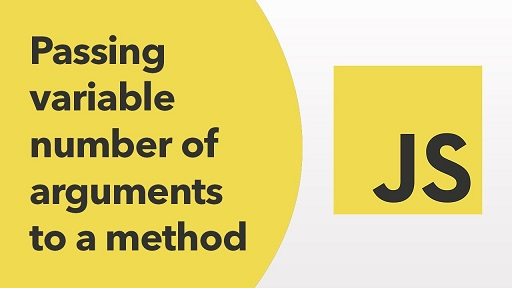# How to Use Variable Number of Arguments in JS Function

Generally, most JavaScript functions accept a fixed number of arguments. But sometimes you may need to use variable number of arguments in JS function. In this article, we will learn how to do this. It is useful if the function caller doesn’t know the number of arguments accepted in function definition, and also if you want to avoid errors due to different number of arguments between function definition and its call.

## How to Use Variable Number of Arguments in JS Function

There are a couple of ways to solve this problem. We will look at each of them one by one.

## 1. Using Arguments Object

By default, every JS function has a special array-like object called arguments that stores all arguments pertaining to the function in which it is referenced. It does not provide all functions available to a general purpose JS array but only length property.

Here is an example to use arguments object to access variable number of arguments.

```function my_func() {
for (var i = 0; i < arguments.length; i++) {
console.log(arguments[i]);
}
}```

In the above function my_func(), we simply loop through arguments object to retrieve all arguments that were passed to the function call.

If you want to convert the above arguments object into a general purpose JS array that offers more features, you can create its copy and use it like any other array. For this purpose, you can use concat() method.

`var args = [].concat.call(arguments)`

You can also use spread operator (…) in function definition to accept a variable number of arguments, as shown below.

```function my_func(...args) {
// args is an Array
console.log(args);
// You can pass this array as parameters to another function
console.log(...args);
}```

In the above function, all arguments passed in function call of my_func() will be stored in array args. You can access them using array name and indexes. You can also pass it to other function calls by prepending it with spread operator (e.g. console.log(…args)). It is also called as rest parameter syntax.

In fact, you can even use it in conjunction with several fixed arguments, as shown below. Here is an example where arguments x, y, and z are fixed while the ones that follow can be variable in number.

```function my_func(x, y, z, ...args) {
// args is an Array
console.log(x, y, z, args);
// You can pass this array as parameters to another function
console.log(...args);
}```

## 3. Using JS object

You can also pass a JS object as argument so that its contents are dynamic in nature. For example, let us say you have the following function definition.

```function load(context)
{
// do something with context.key1, context.key2, etc.
}```

Then you can call the above function as follows.

`load({key1:'Ken',key2:'secret',unused:true})`

If you don’t know the key values present in JS object argument, you can object.keys() to retrieve them.

```function load(context)
{
keys=context.keys();
//do something with context and keys.
}```

In this article, we have learnt a couple of simple ways to use variable number of arguments in JS functions. It is very useful if you want to pass a long list of arguments to a function, with arguments at arbitrary locations. In fact, the second approach above is more suitable for such use cases.